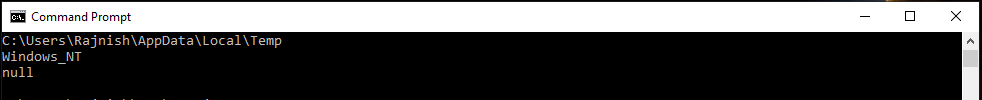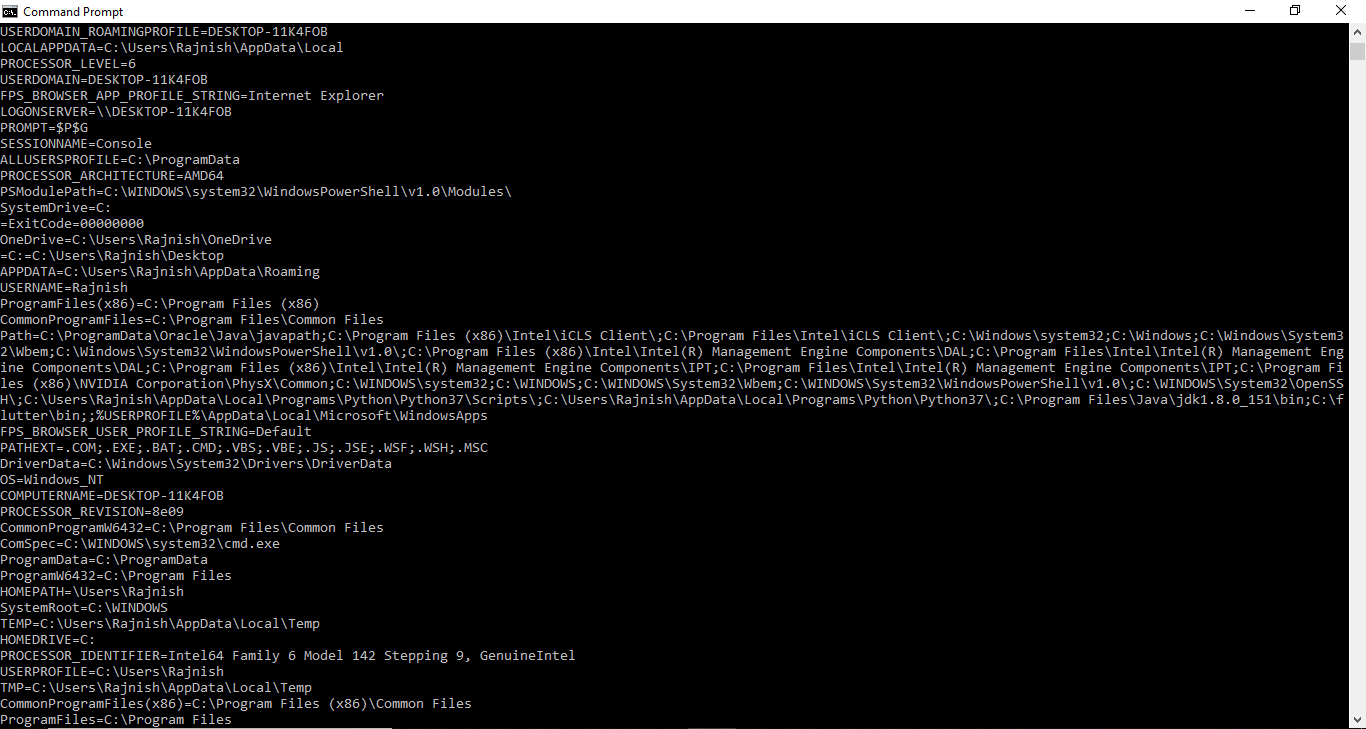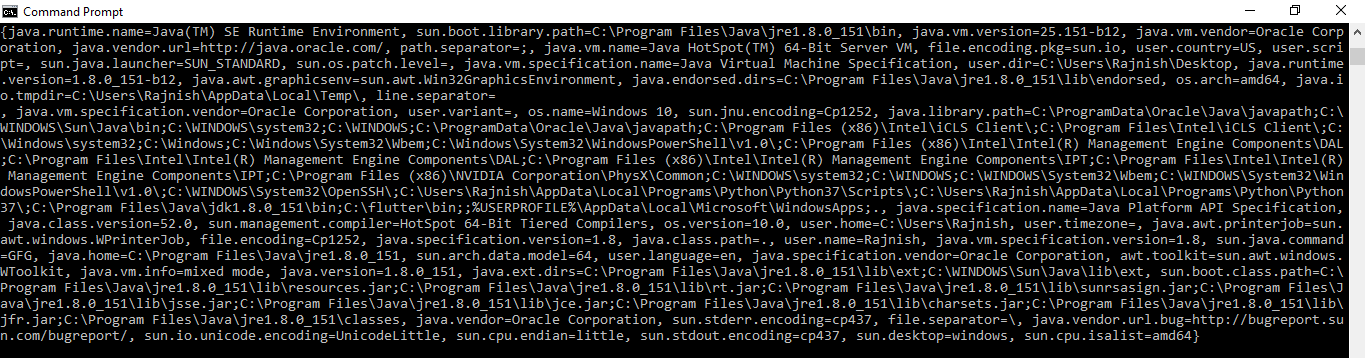GeeksforGeeks App
Open AppBrowser
Continue

# How to get the value of System Property and Environment Variable in Java?

### How to get the value of Environment variables?

The System class in Java provides a method named System.getenv() which can be used to get the value of an environment variable set in the current system.

Syntax:

```public static String getenv(String key);

where key is the Environment variable
whose values we want
```

Below example illustrates how to use System.getenv() to get the System environment variable:

Example 1: To get the value of a specific environment variable

 `// Java program to get the value``// of a specific environment variable``// using System.getenv() method`` ` `public` `class` `GFG {``    ``public` `static` `void` `main(String[] args)``    ``{`` ` `        ``// Get the value of``        ``// the TEMP environment variable``        ``System.out.println(System.getenv(``"TEMP"``));`` ` `        ``// Get the value of``        ``// the OS environment variable``        ``System.out.println(System.getenv(``"OS"``));`` ` `        ``// Get the value of``        ``// the JAVA_HOME environment variable``        ``System.out.println(System.getenv(``"JAVA_HOME"``));``    ``}``}`

Output:Example 2: To get the value of all environment variables at once

 `// Java program to get the value``// of all environment variables at once``// using System.getenv() method`` ` `import` `java.util.Map;`` ` `public` `class` `GFG {``    ``public` `static` `void` `main(String[] args)``    ``{`` ` `        ``// Get the value of``        ``// all environment variables at once``        ``// and store it in Map``        ``Map env``            ``= System.getenv();`` ` `        ``for` `(String envName : env.keySet()) {``            ``System.out.format(``"%s=%s%n"``,``                              ``envName,``                              ``env.get(envName));``        ``}``    ``}``}`

Output:Note: The output will depend on the system on which you run the above code. A sample output is given above

### How to get the value of System Property?

The System class in Java has two methods used to read system properties:

• java.lang.System.getProperty(String key): fetches only those properties – values that you will specify using the key(associated to that particular value that you want).

Example:

 `// Java Program illustrating the working``// of getProperty(String key) method`` ` `import` `java.lang.*;``import` `java.util.Properties;`` ` `public` `class` `NewClass {``    ``public` `static` `void` `main(String[] args)``    ``{``        ``// Printing Name of the system property``        ``System.out.println(``"user.dir: "``                           ``+ System.getProperty(``                                 ``"user.dir"``));`` ` `        ``// Fetches the property set with 'home' key``        ``System.out.println(``"home: "``                           ``+ System.getProperty(``                                 ``"home"``));``        ``// Resulting in Null as no property is present`` ` `        ``// Printing 'name of Operating System'``        ``System.out.println(``"os.name: "``                           ``+ System.getProperty(``                                 ``"os.name"``));`` ` `        ``// Printing 'JAVA Runtime version'``        ``System.out.println(``"version: "``                           ``+ System.getProperty(``                                 ``"java.runtime.version"``));`` ` `        ``// Printing 'name' property``        ``System.out.println(``"name: "``                           ``+ System.getProperty(``                                 ``"name"``));``        ``// Resulting in Null as no property is present``    ``}``}`

Output:

```user.dir: /tmp/hsperfdata_bot
home: null
os.name: Linux
version: 1.8.0_101-b13
name: null
```
• java.lang.System.getProperty(String key, String definition): helps you to create your own key-value sets that you want.

Example:

 `// Java Program illustrating the working of``// getProperty(String key, String definition) method`` ` `import` `java.lang.*;``import` `java.util.Properties;`` ` `public` `class` `NewClass {``    ``public` `static` `void` `main(String[] args)``    ``{`` ` `        ``// Here key = "Hello" and``        ``// System Property = "Geeks"``        ``System.out.println(``"Hello property : "``                           ``+ System.getProperty(``                                 ``"Hello"``, ``"Geeks"``));`` ` `        ``// Here key = "Geek" and``        ``// System Property = "For Geeks"``        ``System.out.println(``"System-property :"``                           ``+ System.getProperty(``                                 ``"System"``, ``"For Geeks"``));`` ` `        ``// Here key = "Property" and``        ``// System Property = null``        ``System.out.println(``"Property-property :"``                           ``+ System.getProperty(``                                 ``"Property"``));``    ``}``}`

Output:

```Hello key property : Geeks
System key property :For Geeks
Property key property :null
```
• java.lang.System.getProperties(): fetches all the properties – values that the JVM on your System gets from the Operating System.

Example:

 `// Java Program illustrating the working of``// getProperties() method`` ` `public` `class` `GFG {``    ``public` `static` `void` `main(String[] args)``    ``{`` ` `        ``System.out.println(System.getProperties())``    ``}``}`

Output:My Personal Notes arrow_drop_up